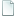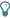Topic: n-tuplets (vs m-tuplets) (Read 24524 times)

## n-tuplets (vs m-tuplets)##### 2003-01-20 04:14 pm
This is an extension of the user tip on Pentuplets and may be helpful when necessary to divide a beat, two beats, or a measure into n parts where n contains more than only 2's or only one 3.  For example, 11 (a prime number other than 2 or 3) would be covered while 12, a product of 2 * 2 * 3, would not be.  Notes can already be divided in half or grouped into triplets so no workaround is necessary.

There are three situations when dealing with n-tuplets.
• Only one part is playing the n-tuplets or all parts are doing the n-tuplets.
• One or more parts are performing n-tuplets while other parts are doing ordinary notes (dotted, double-dotted, or tripletized notes are included), that is, their factors are 2's and/or only one 3.
• One or more parts are performing n-tuplets while other parts are doing m-tuplets where m and n are not equal and m's factors include numbers other than 2's or a 3.

Case 1. is the most straightforward.  Divide n by the next lower power of 2 (1,2,4,8, etc.) and temporarily increase the tempo by that amount for the duration of the n-tuplets.  Hide the tempo changes before and after, beam the n-tuplets (if appropriate), and (optional) insert n as italic text at the middle note.  If there are other staves doing the n-tuplets, these changes would be done there as well except the hidden tempo change only needs to be in one staff.

Case 2. was partially addressed in the pentuplet user tip.  The Case 1. changes would also need to be done for all staves with the n-tuplets, but ordinary notes would need to be extended as well.  To determine by how much, subtract the bottom of the fraction from the top in Case 1.  With 5-tuplets, the time factor is 5/4 and the extension factor would be 1/4.  Tie each note to a copy of itself and add two flags.  With 7-tuplets the time factor is 7/4 and the extension is 3/4.  To the extension note, add one flag and a dot.  To do eleven notes in the space of 8, the time factor is 11/8 and extension is 3/8--add two flags and a dot to the tied notes.  What about fifteen notes in the space of eight?  Since its factors are 3 * 5, it can be broken into five triplets and treated as a pentuplet.

As with the Pentuplet tip, the displayed (but muted) staff would not have the ties. The extension notes would either be hidden or replaced with hidden rests if beaming was not an issue.

Case 3. is the most challenging as both n and m require workarounds.  When done simultaneously, the beat, beats, or measure would need to be broken into the Lowest Common Multiple (LCM) of n and m.  If both n and m are prime or share no common factors then they would be multiplied to obtain the LCM.  For example, if a measure contained five notes being played with seven notes concurrently in another part, the measure would have to contain thirty-five(!) beats.  Each note of the pentuplet would use seven of these (one double-dotted note per each would suffice) while the septuplet would require five beats each.

Sometimes n and m would have a common factor or a factor which can be handled by the notation already.  For example, to have 7 vs. 9 in a measure, it would not be necessary to put 63 beats in a measure (although that would work).  Treat it as 7 vs. 3 (21) instead and the part(s) with the 9 can be grouped into three sets of triplets.

What about 6 vs 7 vs 8 vs 9?  While I doubt any music would ever contain this, its LCM would be 2 * 3 * 7 * 2 * 2 * 3 or 504.  However, the 2's and one 3 would go away leaving only 21 beats.  The combination of adding flags (halving the note) or creating triplets would handle all of these divisions.  A much simpler example is 6 vs 9.  Only one 3 needs to be accounted for so, with a time signature of 3/4, triplets are played against eighth notes and no workaround is needed.

In creating a demonstration of 1-10 beats to a bar simultaneously, the LCM of those notes would include a number of 2's, two 3's, a 5 and a 7.  The 2's go away as does one of the 3's as shown in the previous paragraph, leaving a time signature of 3 * 5 * 7 = 105.

Another related user tip is Triplets v. Duples, 3/4 v. 9/8, Hiding "3" in triplets.

This line is added to help searches: pentuplet quintuplet quintuple pentuple n-tuple n-tuplet hextuple hextuplet septuplet septuple.
Since 1998